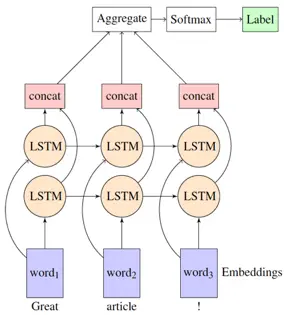# RNN/LSTM-递归神经网络

### 一、RNN vs LSTM

#### RNN 逻辑结构图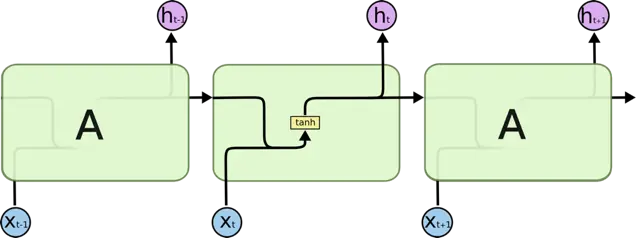#### LSTM 逻辑结构图LSTM 是 RNN 的变体，它们的原理几乎一样，唯一的不同是 output 即 hidden state 的计算逻辑不同

#### RNN 如何计算某个时刻 t 的 output ?

$h_t = tanh(W*[h_{t-1}, x_t]+b)$

#### LSTM 如何计算某个时刻 t 的 output ?

$f_t = sigmoid(W_f*[h_{t-1}, x_t] + b_f)$

${C}^{*} = tanh(W_c*[h_{t-1}, x_t]+b_c)$

$i_t = sigmoid(W_i*[h_{t-1}, x_t] + b_i)$

$C_t = f_t*C_{t-1} + i_t*{C}^{*}$

$o_t = sigmoid(W_o*[h_{t-1}, x_t] + b_o)$

$h_t = o_t*tanh(C_t)$

#### LSTM 为什么优于 RNN ?

RNN 通过叠乘的方式进行状态更新，当 sequence 比较长时容易出现梯度消失/爆炸的情况，主要原因是反向传播的连乘效应，而 LSTM 是通过门控制的叠加方式来更新状态（C_t的计算公式），所以可以有效防止梯度问题，当然对于超长的 sequence，LSTM 依然会有梯度消失或者爆炸的可能。

### 二、RNN/LSTM 中的 num_units 是啥意思 ？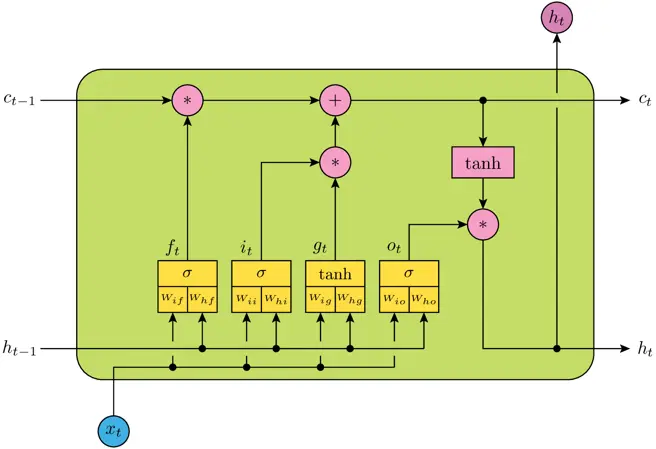num_units 相当于神经网络的隐层神经元的个数，例如上图表示一个 LSTM Cell，包含四个神经网络层，即黄色方框部分，num_units 就是每个神经网络结构的隐层神经元个数(全连接单元数)，它实际上也是 LSTM 输出向量的维度数，所以 h_t 为 num_units 维向量。

### 三、如何计算 Keras 的 LSTM layer 的参数个数？

$(num\_units + input\_dims + 1) * num\_units * 4 = 150600$

1. num_units + input_dims 是因为上层输出需要首先与输入进行一次concat，即 [h_t-1, x_t],
2. + 1 是因为 bias
3. * 4 是因为共有4个神经网络层（黄色方框）
4. 为什么不需要乘以 time_steps 即 number of cell ?

### 五、为什么 LSTM 的输入和输出值不用 sigmoid 而用 tanh 作为激活函数 ？

LSTM 内部维护了一个状态向量，其值应该可以增加或者减少，而 sigmoid 的输出为非负数，所以状态信息只能增加，显然不合适，相反，tanh 的输出范围包含了正负数，因此可以满足状态的增减。

[1,2,3,4] => 5
[2,3,4,5] => 6

### 七、Keras 中的 return_sequence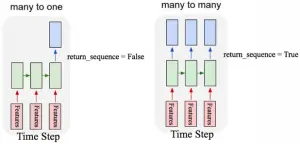### 八、Keras 中 LSTM 的 stateful 概念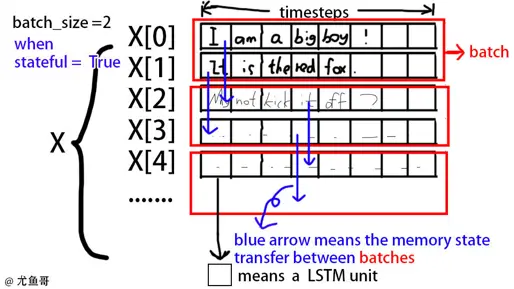### 九、Keras 中 LSTM 的 state 何时 reset?

LSTM 的 state 是针对每一个 sequence 而言的，所以对于一个 batch_size = 10 的 batch，它会为10个 sequences 同时创建10个并行的 state。

### 十、常用 LSTM 模型结构

#### 多层 LSTM#### 双向 LSTM